# Minimization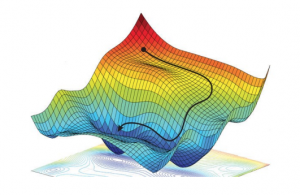The training (also known as learning or optimisation phase) of neural networks is carried out using the gradient descent method in one of its versions such as back-propagation or stochastic gradient descent. In these methods, the determination of the fit parameters (namely the weights and thresholds of the NN) requires the evaluation of the gradients of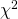, that is,

(1)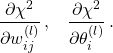Computing these gradients in the NNPDF case involves handling the non-linear relation between the fitted experimental data and the input PDFs, which proceeds through convolutions both with the DGLAP evolution kernels and the hard-scattering partonic cross-sections as encoded into the optimised APFELgrid fast interpolation strategy.

The theory prediction for a collider cross-section in terms of the NN parameters reads

(2)where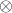indicates a convolution over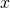,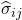andstand for the hard-scattering cross-sections and the DGLAP evolution kernels respectively, and sum over repeated flavour indices is understood.

In the APFELgrid approach, this cross-section can be expressed in a much compact way as

(3)where now all perturbative information is pre-computed and stored in theinterpolation tables, and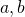run over a grid in.